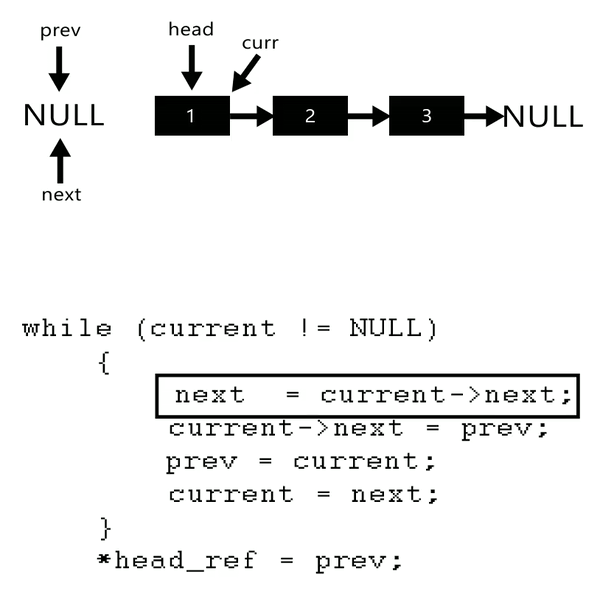84 views
Give a $\Theta(n)$ time nonrecursive procedure that reverses a singly linked list of $n$ elements. The procedure should use no more than constant storage beyond that needed for the list itself.
| 84 views

Iterative Method

1. Initialize three pointers prev as NULL, curr as head and next as NULL.
2. Iterate trough the linked list. In loop, do following.
// Before changing next of current,
// store next node
next = curr->next

// Now change next of current
// This is where actual reversing happens
curr->next = prev

// Move prev and curr one step forward
prev = curr
curr = next// Iterative C program to reverse a linked list
#include <stdio.h>
#include <stdlib.h>

struct Node {
int data;
struct Node* next;
};

/* Function to reverse the linked list */
{
struct Node* prev = NULL;
struct Node* next = NULL;
while (current != NULL) {
// Store next
next = current->next;

// Reverse current node's pointer
current->next = prev;

// Move pointers one position ahead.
prev = current;
current = next;
}
}

/* Function to push a node */
void push(struct Node** head_ref, int new_data)
{
struct Node* new_node = (struct Node*)malloc(sizeof(struct Node));
new_node->data = new_data;
}

/* Function to print linked list */
{
while (temp != NULL) {
printf("%d  ", temp->data);
temp = temp->next;
}
}

/* Driver program to test above function*/
int main()
{

}